# 基于Python的OpenCV图像处理11

## May 30,2017   18535 words   67 min

### 图像金字塔

• 1.利用低通滤波器进行图像平滑
• 2.对平滑后的图像进行重采样

• 高斯金字塔(Gaussian pyramid): 用来向下采样，主要的图像金字塔
• 拉普拉斯金字塔(Laplacian pyramid): 用来从金字塔低层图像重建上层未采样图像，在数字图像处理中也即是预测残差，可以对图像进行最大程度的还原，配合高斯金字塔一起使用。

#### 一、高斯金字塔

##### 1.原理与定义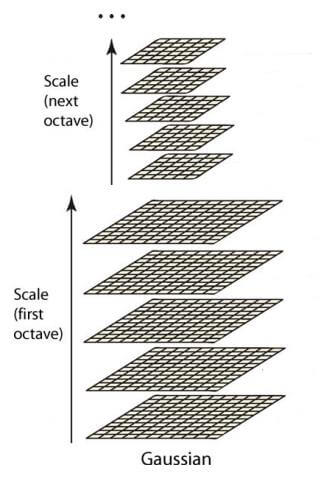###### 尺度空间

$\sigma =\sigma_{0}2^{o+\frac{s}{S}}$

s表示某个八度中某一图像的层数，S表示某个八度的层数，o表示第几个八度。 这里的sigma可以理解为表示图像尺度的变量。其实也就是高斯卷积函数中的一个变量，高斯卷积函数如下表示：

$G(x,y)=\frac{1}{2\pi\sigma^{2}}e^{-\frac{(x-x_{0})^2+(y-y_{0})^2}{2\sigma^{2}}}$

$k=2^{\frac{1}{S}}$

$\sigma = \sigma_{0}2^{o}k^{s}$

sigma0表示图像的初始尺度。这里k只与每个八度中的层数有关。高斯金字塔中每个八度有S+3幅高斯图像， 而关于如何确定S，需要根据实际需求确定。所以每增加一级八度，o加1，所以sigma都要扩大2倍，在一个八度中k的上标s用来 区分不同的高斯核。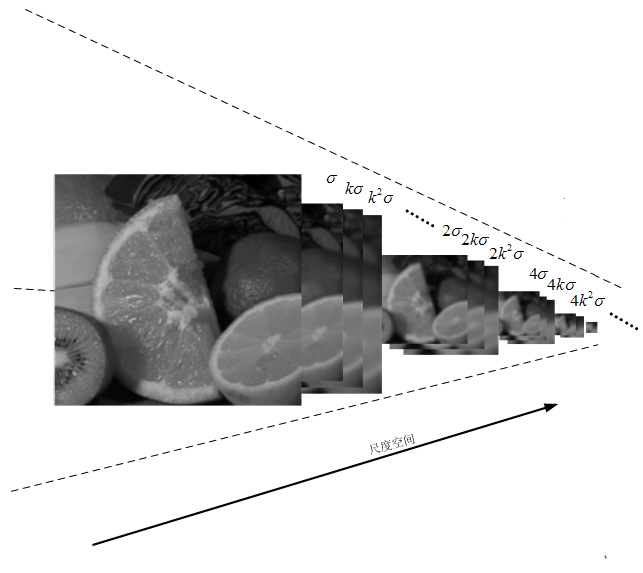这样，我们令sigma0为δ，o和s都是从0开始。那么由上面的公式可以得到对于第一个八度依次有：

$\delta,k\delta,k^{2}\delta,k^{3}\delta...$

$2\delta,2k^{2}\delta,2k^{3}\delta,2k^{4}\delta...$

##### 2.高斯差分金字塔(DOG)

$L(x,y,\sigma)=D(x,y,\sigma)\otimes I(x,y)$

$D(x,y,\sigma)\\=(D(x,y,k\sigma)-D(x,y,\sigma))\otimes I(x,y)\\=L(x,y,k\sigma)-L(x,y,\sigma)$

L(x,y,σ)表示某一层，所以这个公式就表示了上一层与下一层做差，即可得到DOG。

##### 3.尺度空间连续性

$k=2^{\frac{1}{S}}$

$\delta,2^{\frac{1}{3}}\delta,2^{\frac{2}{3}}\delta,2^{\frac{3}{3}}\delta,2^{\frac{4}{3}}\delta,2^{\frac{5}{3}}\delta$

$\delta,2^{\frac{1}{3}}\delta,2^{\frac{2}{3}}\delta,2^{\frac{3}{3}}\delta,2^{\frac{4}{3}}\delta$

$2\times \delta,2\times2^{\frac{1}{3}}\delta,2\times2^{\frac{2}{3}}\delta,2\times2^{\frac{3}{3}}\delta,2\times2^{\frac{4}{3}}\delta,2\times2^{\frac{5}{3}}\delta$

$2\times\delta,2\times2^{\frac{1}{3}}\delta,2\times2^{\frac{2}{3}}\delta,2\times2^{\frac{3}{3}}\delta,2\times2^{\frac{4}{3}}\delta$

$2^{\frac{1}{3}}\delta,2^{\frac{2}{3}}\delta,2^{\frac{3}{3}}\delta,2\times2^{\frac{1}{3}}\delta,2\times2^{\frac{2}{3}}\delta,2\times2^{\frac{3}{3}}\delta$

$2^{\frac{1}{3}}\delta,2^{\frac{2}{3}}\delta,2^{\frac{3}{3}}\delta,2^{\frac{4}{3}}\delta,2^{\frac{5}{3}}\delta,2^{\frac{6}{3}}\delta$

##### 4.高斯金字塔构建步骤
• 1.先将原图像扩大一倍之后作为高斯金字塔的第1组第1层，将第1组第1层图像经高斯卷积（其实就是高斯平滑或称高斯滤波） 之后作为第1组金字塔的第2层。
• 2.将σ乘以一个比例系数k,等到一个新的平滑因子σ=k*σ，用它来平滑第1组第2层图像，结果图像作为第3层。
• 3.如此这般重复，最后得到L层图像，在同一组中，每一层图像的尺寸都是一样的，只是平滑系数不一样。 它们对应的平滑系数分别为：0，σ，kσ，k^2σ,k^3σ……k^(L-2)σ。
• 4.将第1组倒数第三层图像作比例因子为2的降采样，得到的图像作为第2组的第1层， 然后对第2组的第1层图像做平滑因子为σ的高斯平滑，得到第2组的第2层，就像步骤2中一样， 如此得到第2组的L层图像，同组内它们的尺寸是一样的，对应的平滑系数分别为：0，σ，kσ，k^2σ,k^3σ……k^(L-2)σ。 但是在尺寸方面第2组是第1组图像的一半。

#### 二、拉普拉斯金字塔

$L_{i}=G_{i}-UP(G_{i+1})\otimes G_{5\times 5}$

$L_{i}=G_{i}-PyrUp(G_{i+1})$

#### 三、OpenCV实现

##### 1.高斯金字塔

# coding=utf-8
import cv2

gauss1 = cv2.pyrDown(gauss0)
gauss2 = cv2.pyrDown(gauss1)
gauss3 = cv2.pyrDown(gauss2)

cv2.imshow("0", gauss0)
cv2.imshow("1", gauss1)
cv2.imshow("2", gauss2)
cv2.imshow("3", gauss3)
cv2.waitKey(0)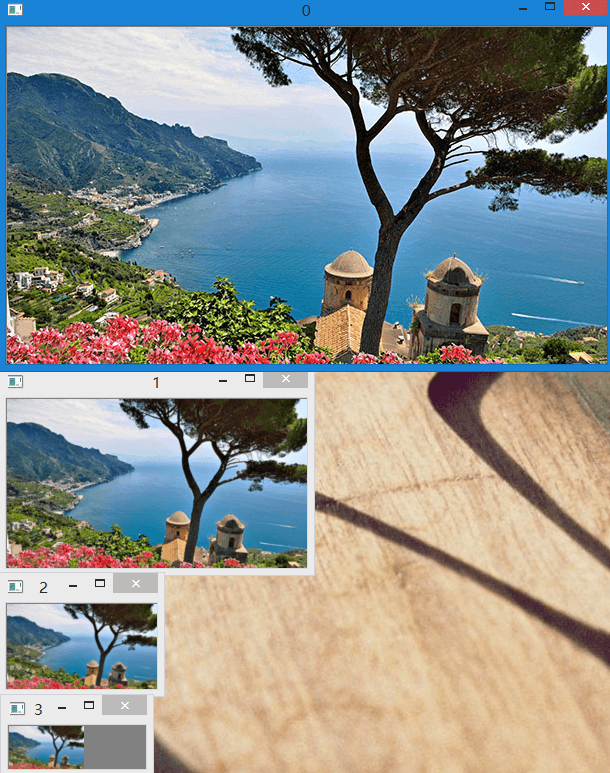##### 2.拉普拉斯金字塔

# coding=utf-8
import cv2

def getLplacian(gauss, g):
gauss_width = gauss.shape
gauss_height = gauss.shape
g_width = g.shape
g_height = g.shape
d_width = g_width - gauss_width
d_height = g_height - gauss_height
if d_width != 0 or d_height != 0:
temp = cv2.copyMakeBorder(gauss, d_width, 0, d_height, 0, cv2.BORDER_REPLICATE)
else:
temp = gauss
layer = cv2.subtract(temp, g)
return layer

# 首先生成高斯金字塔
gauss1 = cv2.pyrDown(gauss0)
gauss2 = cv2.pyrDown(gauss1)
gauss3 = cv2.pyrDown(gauss2)

# 然后利用上采样得到上一层图像
g2 = cv2.pyrUp(gauss3)
g1 = cv2.pyrUp(gauss2)
g0 = cv2.pyrUp(gauss1)

# 最后将对应层做差
# 注意对两个图像做差的时候，不要直接减，那会使用numpy的矩阵计算，
# 减出来的结果是不对的
l0 = getLplacian(gauss0, g0)
l1 = getLplacian(gauss1, g1)
l2 = getLplacian(gauss2, g2)

# 显示拉普拉斯金字塔
cv2.imshow("0", l0)
cv2.imshow("1", l1)
cv2.imshow("2", l2)

# 显示高斯金字塔
cv2.imshow("g0", gauss0)
cv2.imshow("g1", gauss1)
cv2.imshow("g2", gauss2)
cv2.waitKey(0)


###### (1)重采样图片大小问题

def getLplacian(gauss, g):
gauss_width = gauss.shape
gauss_height = gauss.shape
g_width = g.shape
g_height = g.shape
d_width = g_width - gauss_width
d_height = g_height - gauss_height
if d_width != 0 or d_height != 0:
temp = cv2.copyMakeBorder(gauss, d_width, 0, d_height, 0, cv2.BORDER_REPLICATE)
else:
temp = gauss
layer = cv2.subtract(temp, g)
return layer


1.直接相减

2.修改加法参数

3.使用减法

#### 四、图像金字塔应用实例

• 1.读入两幅图像
• 2.构建苹果和桔子的高斯金字塔
• 3.根据高斯金字塔计算拉普拉斯金字塔
• 4.在拉普拉斯金字塔的每一层进行图像融合
• 5.根据融合后的图像金字塔重建原始图像

# coding=utf-8
import numpy as np
import cv2

gauss_width = gauss.shape
gauss_height = gauss.shape
g_width = g.shape
g_height = g.shape
d_width = g_width - gauss_width
d_height = g_height - gauss_height
if d_width != 0 or d_height != 0:
temp = cv2.copyMakeBorder(gauss, d_width, 0, d_height, 0, cv2.BORDER_REPLICATE)
else:
temp = gauss
return layer

def getLplacianSub(gauss, g):
gauss_width = gauss.shape
gauss_height = gauss.shape
g_width = g.shape
g_height = g.shape
d_width = g_width - gauss_width
d_height = g_height - gauss_height
if d_width != 0 or d_height != 0:
temp = cv2.copyMakeBorder(gauss, d_width, 0, d_height, 0, cv2.BORDER_REPLICATE)
else:
temp = gauss
layer = cv2.subtract(temp, g)
return layer

# 注意，这里我们对图像进行裁剪，不能使用图像缩放resize。
# 因为我们在采样的过程中，根据我们的算法，是在top和left两个方向增加了数值
# 所以整体图像是像右下方移动的
# 所以我们需要把我们重采样过程中top和left方向上多加的像素给减掉
# 如果只是单纯的resize，会发现图片整体和原图虽然大小一致，
# 但是图片中物体的位置是移动了的
# 因此这个操作是图像裁剪，与我们重采样时扩边操作对应
# 而不是图像的缩放
def restoreXY(origin, present):
ori_row = origin.shape
ori_col = origin.shape
pre_row = present.shape
pre_col = present.shape
if ori_row != pre_row or ori_col != pre_col:
result = present[pre_row - ori_row:, pre_col - ori_col:]
else:
result = present
return result

gpL = 6

# 为苹果建立高斯金字塔，包括原图共7层
gpA = [apple]
for i in range(gpL):
g = cv2.pyrDown(gpA[i])
gpA.append(g)

# 为桔子建立高斯金字塔，包括原图共7层
gpO = [orange]
for i in range(gpL):
g = cv2.pyrDown(gpO[i])
gpO.append(g)

# 基于高斯金字塔建立苹果的拉普拉斯金字塔
# 注意这里在建完金字塔后，将金字塔的第一层
# 用高斯金字塔的倒数第二层(第五层)替代了
# 这样才能再后来重新上采样的时候还原原图
# 否则只靠拉普拉斯金字塔的信息上采样是无法
# 还原原图的，只会得到黑乎乎的图像
# 而之所以用第5层，是因为建拉普拉斯金字塔
# 只能从高斯金字塔的导数第二层建起，而
# 倒数第二层对应索引是5
# 当然其实还有一种方案，那就是不替代拉普拉斯金字塔
# 的第一层，而是在第一层的前面再加一层高斯
# 金字塔。这样得到的拉普拉斯金字塔一共是7层
# 包括一层高斯金字塔的最后一层和6层正常的拉普拉斯
# 金字塔。但这样可能会给后面的范围带来些麻烦，
# 而且多一层对于融合效果提升不大，所以这里不采用这个方法。
# 当然可能会好奇，为什么一定要用
# 高斯金字塔的最后一层。前面也说了，正是因为
# 需要恢复原图信息，必须要有原图，否则是无法还原
# 原图信息的。高斯金字塔包含原图信息。
lpA = []
for i in range(gpL, 0, -1):
l = getLplacianSub(gpA[i - 1], cv2.pyrUp(gpA[i]))
lpA.append(l)
lpA = gpA[-2]

# 基于高斯金字塔建立桔子的拉普拉斯金字塔
# 同时注意拉普拉斯金字塔的第一层的替换
lpO = []
for i in range(gpL, 0, -1):
l = getLplacianSub(gpO[i - 1], cv2.pyrUp(gpO[i]))
lpO.append(l)
lpO = gpO[-2]

# 对于每一层金字塔将两个图像各取一半合并
# 这里用到了Numpy的hstack函数，表示将两个矩阵合并到一起，注意不是加
# 其中h表示水平方向，v表示竖直方向
# 例如A为3*3，B为3*3，hstack合并后矩阵大小是3*6
lpAO = []
for i in range(gpL):
rows, cols, bpt = lpA[i].shape
lp = np.hstack((lpA[i][:, :cols / 2, :], lpO[i][:, cols / 2:, :]))
lpAO.append(lp)

# 逐步上采样重建金字塔
# 注意这里的迭代变量关系
# 这里是拉普拉斯金字塔第一层上采样到与第二层大小一致，
# 然后与现有的第二层相加，得到新的第二层
# 再将这个新的第二层上采样，和第三层相加
# 所以需要注意并不是拿老的第二层与第三层相加
# 否则是得不到正确结果的
gao = lpAO
for i in range(gpL - 1):
gao = cv2.pyrUp(gao)
gao = getLplacianAdd(lpAO[i + 1], gao)

# 由于重采样回来后图像变大了，所以可以重新裁剪到原图大小
gao = restoreXY(apple, gao)

# 直接混合对比
direct = np.hstack((apple[:, :apple.shape / 2], orange[:, orange.shape / 2:]))

cv2.imshow("gao" + gpL.__str__(), gao)
cv2.imshow("direct", direct)
cv2.waitKey(0)


##### 3.拉普拉斯金字塔建立

###### (2)疑惑二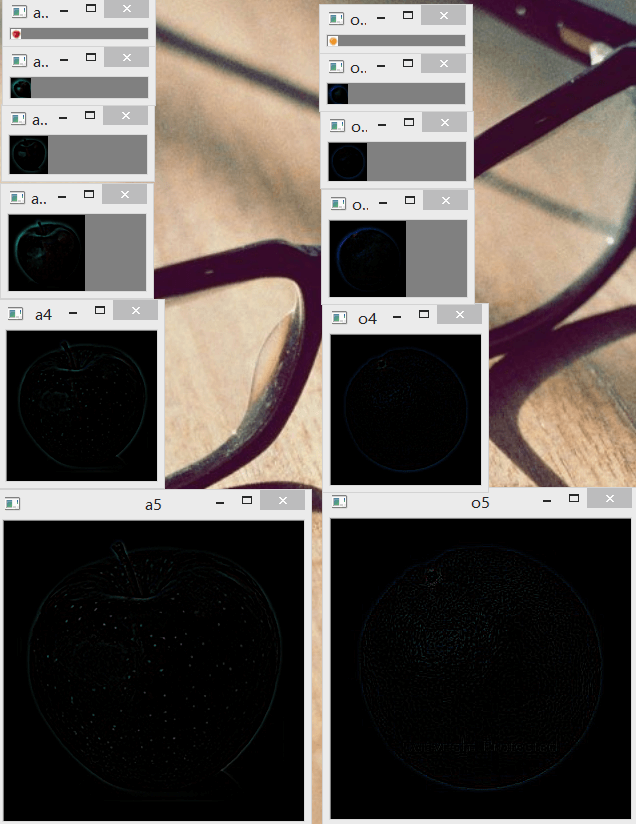###### (4)疑惑四

$G_{i}=L_{i}+PyrUp(G_{i+1})$

##### 6.图像压缩

# coding=utf-8
import cv2

gauss_width = gauss.shape
gauss_height = gauss.shape
g_width = g.shape
g_height = g.shape
d_width = g_width - gauss_width
d_height = g_height - gauss_height
if d_width != 0 or d_height != 0:
temp = cv2.copyMakeBorder(gauss, d_width, 0, d_height, 0, cv2.BORDER_REPLICATE)
else:
temp = gauss
return layer

def getLplacianSub(gauss, g):
gauss_width = gauss.shape
gauss_height = gauss.shape
g_width = g.shape
g_height = g.shape
d_width = g_width - gauss_width
d_height = g_height - gauss_height
if d_width != 0 or d_height != 0:
temp = cv2.copyMakeBorder(gauss, d_width, 0, d_height, 0, cv2.BORDER_REPLICATE)
else:
temp = gauss
layer = cv2.subtract(temp, g)
return layer

def restoreXY(origin, present):
ori_row = origin.shape
ori_col = origin.shape
pre_row = present.shape
pre_col = present.shape
if ori_row != pre_row or ori_col != pre_col:
result = present[pre_row - ori_row:, pre_col - ori_col:]
else:
result = present
return result

# 显示原图
cv2.imshow("standard", apple)

# 将OpenCV读取的图像保存重新保存一下作为参照
# 注意不应该拿原始图像与经过OpenCV重新保存的图像比较大小
# 因为压缩算法，保存质量等原因会有很大差别
# 因此应该先将读取的图片保存一下作为参照
# 后续金字塔压缩的图片与这个图片比较大小
cv2.imwrite("E:\\out\\standard.jpg", apple)

# 建立金字塔的层数
gpL = 4

# 为读取的图像建立金字塔(gpL+1)层金字塔，其中第一层为原图
gpA = [apple]
for i in range(gpL):
g = cv2.pyrDown(gpA[i])
gpA.append(g)

# 根据建立的(gpL+1)层高斯金字塔生成gpL层拉普拉斯金字塔
# 同时为了后期能对图像进行还原，和融合一样，将第一层
# 用高斯金字塔的倒数第二层替换掉
lpA = []
for i in range(gpL, 0, -1):
l = getLplacianSub(gpA[i - 1], cv2.pyrUp(gpA[i]))
lpA.append(l)
lpA = gpA[-2]

# 遍历金字塔，显示、输出各层图像
for i in range(gpL):
cv2.imshow(i.__str__(), lpA[i])
cv2.imwrite('E:\\out\\' + i.__str__() + '.jpg', lpA[i])

# 利用金字塔进行图像重建，测试效果
gao = lpA
for i in range(gpL - 1):
gao = cv2.pyrUp(gao)
gao = getLplacianAdd(lpA[i + 1], gao)

# 修改因扩边操作增加的大小
gao = restoreXY(apple, gao)

# 显示利用金字塔恢复的图像
cv2.imshow("gao" + gpL.__str__(), gao)
cv2.waitKey(0)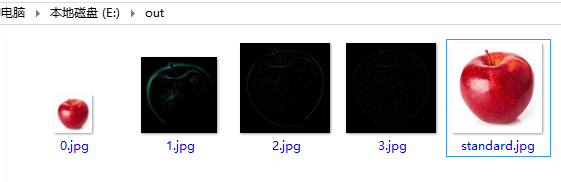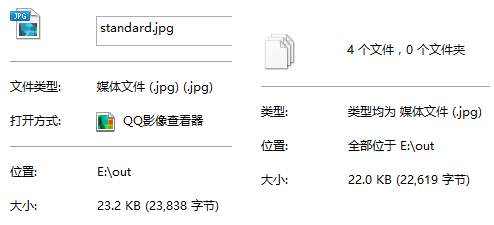同时，我们还可以研究统计不同金字塔层数对于压缩大小的影响，如下表所示。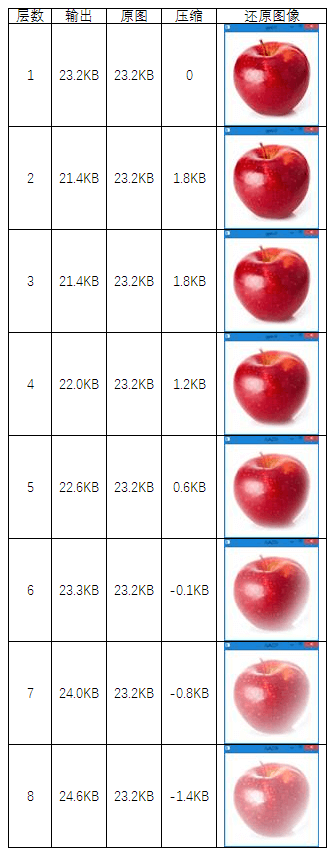可以发现如下几条规律：

• 1.压缩率与金字塔层数有关系，但并不是层数越多压缩越多，而是有一个最佳的阈值。
• 2.与前面说过的一样，层数越多其颜色就越淡，恢复的效果就越差。
• 3.为了兼顾压缩和复原图像质量，应该根据图像合理选择金字塔层数。